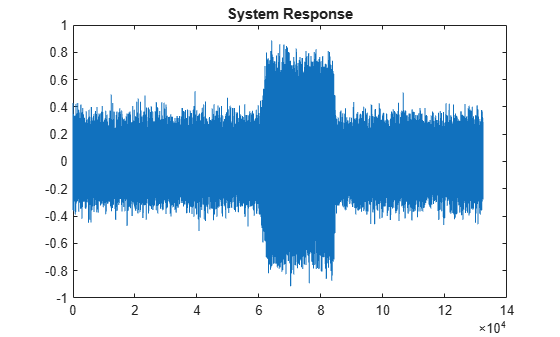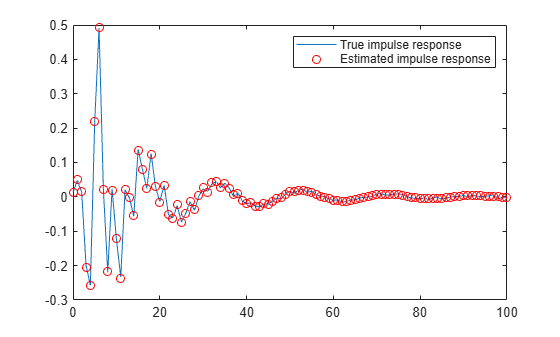# sweeptone

Exponential swept sine

## Syntax

``excitation = sweeptone()``
``excitation = sweeptone(swDur)``
``excitation = sweeptone(swDur,silDur)``
``excitation = sweeptone(swDur,silDur,fs)``
``excitation = sweeptone(___,Name,Value)``

## Description

````excitation = sweeptone()` returns an excitation signal generated using the exponential swept sine (ESS) technique. By default, the signal has a 6-second duration, followed by 4 seconds of silence, for a sample rate of 44100 Hz.```
````excitation = sweeptone(swDur)` specifies the duration of the exponential swept sine signal.```
````excitation = sweeptone(swDur,silDur)` specifies the duration of the silence following the exponential swept sine signal.```

example

````excitation = sweeptone(swDur,silDur,fs)` specifies the sample rate of the sweep tone as `fs` Hz.```

example

````excitation = sweeptone(___,Name,Value)` specifies options using one or more `Name,Value` pair arguments, in addition to the input arguments in the previous syntaxes.```

## Examples

collapse all

Create a sweep tone excitation signal by using the `sweeptone` function.

```excitation = sweeptone(2,1,44100); plot(excitation) title('Excitation')```Pass the excitation signal through an infinite impulse response (IIR) filter and add noise to model a real-world recording (system response).

```[B,A] = butter(10,[.1 .7]); rec = filter(B,A,excitation); nrec = rec + 0.12*randn(size(rec)); plot(nrec) title('System Response')```Pass the excitation signal and the system response to the `impzest` function to estimate the impulse response. Truncate the estimate to 100 points. Use `impz` to determine the true impulse response of the system. Plot the true impulse response and the estimated impulse response for comparison.

```irEstimate = impzest(excitation,nrec); irEstimate = irEstimate(1:101); irTrue = impz(B,A,101); plot(0:100,irEstimate, ... 0:100,irTrue,'ro') legend('True impulse response','Estimated impulse response')```Generate an exponential swept sine (ESS) signal with a 3-second sweep that goes from 20 Hz to 20 kHz, and ends with a 2-second silence. Specify the sample rate as 48 kHz.

```fs = 48e3; excitation = sweeptone(3,2,fs,'SweepFrequencyRange',[20 20e3]);```

Visualize the excitation in time and time-frequency.

```t = (0:numel(excitation)-1)/fs; plot(t,excitation) xlabel('Time (s)')````spectrogram(excitation,512,0,1024,fs,'yaxis')`## Input Arguments

collapse all

Duration of exponential swept sine signal in seconds, specified as a scalar in the range [0.5,15].

The total duration of the excitation signal must be less than or equal to 15 seconds: `swDur` + `silDur` ≤ 15.

Data Types: `single` | `double` | `int8` | `int16` | `int32` | `int64` | `uint8` | `uint16` | `uint32` | `uint64`

Duration of silence after exponential swept sine, specified as a scalar in the range (0,14.5].

The total duration of the excitation signal must be less than or equal to 15 seconds: `swDur` + `silDur` ≤ 15.

Data Types: `single` | `double` | `int8` | `int16` | `int32` | `int64` | `uint8` | `uint16` | `uint32` | `uint64`

Sample rate in Hz, specified as a positive scalar.

Data Types: `single` | `double` | `int8` | `int16` | `int32` | `int64` | `uint8` | `uint16` | `uint32` | `uint64`

### Name-Value Pair Arguments

Specify optional comma-separated pairs of `Name,Value` arguments. `Name` is the argument name and `Value` is the corresponding value. `Name` must appear inside quotes. You can specify several name and value pair arguments in any order as `Name1,Value1,...,NameN,ValueN`.

Example: `'ExcitationLevel',-5`

Level of the excitation signal to generate in dB, specified as a scalar in the range `[-42,0]`.

Data Types: `single` | `double` | `int8` | `int16` | `int32` | `int64` | `uint8` | `uint16` | `uint32` | `uint64`

Range of sweep frequency in Hz, specified as a two-element row vector. The sweep frequency range can be specified low to high or high to low. That is, ```[10 22000]``` and `[22000 10]` are both valid inputs. The largest value of the sweep frequency range must be less than or equal to `fs`/2.

Data Types: `single` | `double` | `int8` | `int16` | `int32` | `int64` | `uint8` | `uint16` | `uint32` | `uint64`

## Output Arguments

collapse all

Excitation signal generated using the ESS technique, returned as a column vector. The length of the column vector is approximately (`swDur`+`silDur`)*`fs` samples.

Data Types: `double`

 Farino, Angelo. "Advancements in Impulse Response Measurements by Sine Sweeps." Presented at the Audio Engineering Society 122nd Convention, Vienna, Austria, 2007.

## SupportGet trial now